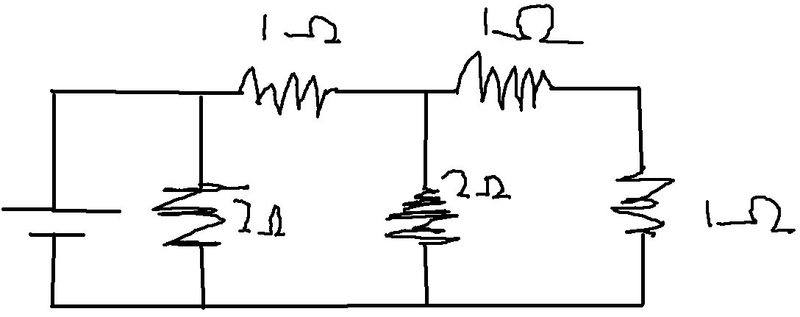# Calculating total resistance

## Homework Statement

I am asked to calculate the total resistance of this circuit## Homework Equations

1/rt =1/r1+1/r2+1/r3....etc - parallel
RT=r1+r2+r3...etc - series

## The Attempt at a Solution

1/rt = 1/2 +1/2 +1/1
rt=1/2
then i tried adding 2 to it (from the top two resistors added together in series i think...)
rt=2.5

I can't follow your post but let's start from the right and with some basics.

If the same current flows through two resistors, they are in series.

If the same voltage is across two resistors, they are in parallel.

Current splits when it flows into a node.

Now, at the right hand side, we have a a 1 ohm and 1 ohm resistor. Does the same current flow through them?

Yes, meaning they are in series. Now, this 2 ohm resistor and the 2 ohm resistor. There is a current coming in from the left hand branch, which splits between the 2 ohm and 2 ohm resistor, meaning they are not in series. Add these two in parallel.

Try and finish the rest of the question yourself, using these basics. If you've any questions ask.